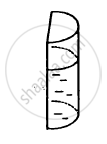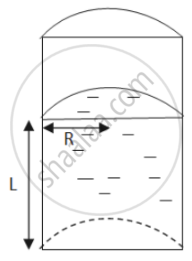Department of Pre-University Education, KarnatakaPUC Karnataka Science Class 11
Advertisement Remove all ads

# A Liquid is Contained in a Vertical Tube of Semicircular Cross Section. the Contact Angle is Zero. the Force of Surface Tension on the Curved Part and on the Flat Part Are in Ratio - Physics

MCQ

A liquid is contained in a vertical tube of semicircular cross section. The contact angle is zero. The force of surface tension on the curved part and on the flat part are in ratio#### Options

• 1:1

•  1:2

• π:2

• 2:π

Advertisement Remove all ads

#### SolutionLet the height of the liquid-filled column be L.
Let the radius be denoted by R.

$\text{ Total perimeter of the curved part = semi - circumference of upper area }= \pi r$

$\text{ Total surface tension force } = \pi RS$

$\text{ Total perimeter of the flat part = 2R }$

$\text{ Total surface tension force = 2RS }$

$\text{ Ratio of curved surface force to flat surface force } = \frac{\pi RS}{2RS} = \frac{\pi}{2}$



Is there an error in this question or solution?
Advertisement Remove all ads

#### APPEARS IN

HC Verma Class 11, 12 Concepts of Physics 1
Chapter 14 Some Mechanical Properties of Matter
MCQ | Q 5 | Page 299
Advertisement Remove all ads

#### Video TutorialsVIEW ALL 

Advertisement Remove all ads
Share
Notifications

View all notifications

Forgot password?